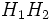Normal coalition class

This article defines a group metaproperty: a property that can be evaluated to true/false for any group property
View a complete list of group metaproperties

Contents

This article defines a term that has been used or referenced in a journal article or standard publication, but may not be generally accepted by the mathematical community as a standard term.[SHOW MORE]

History

Origin of the term

The term normal coalition class was introduced by J.E. Roseblade in the paper On Certain Subnormal Coalition Classes in Journal of Algebra, I, 132-138 (1964).

Definition

Definition with symbols

A group property$p$ of groups is said to give a normal coalition class if whenever$G$ is a group and$H_1$ and$H_2$ are normal subgroups of$G$ both satisfying$p$ as groups, then the subgroup$H_1H_2$ also satisfies$p$.